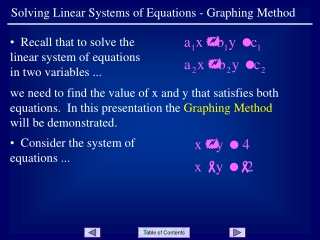DownloadDownload PresentationSolving Linear Systems of Equations - Graphing Method

# Solving Linear Systems of Equations - Graphing Method

Download Presentation## Solving Linear Systems of Equations - Graphing Method

- - - - - - - - - - - - - - - - - - - - - - - - - - - E N D - - - - - - - - - - - - - - - - - - - - - - - - - - -
##### Presentation Transcript

1. Solving Linear Systems of Equations - Graphing Method • Recall that to solve the linear system of equations in two variables ... we need to find the value of x and y that satisfies both equations. In this presentation the Graphing Method will be demonstrated. • Consider the system of equations ...

2. Solving Linear Systems of Equations - Graphing Method • Sketch a graph of the first equation ... • Each point on the graph of the function satisfies the equation of the function ... • (x,y) Slide 2

3. Solving Linear Systems of Equations - Graphing Method • Now add a sketch of the second function to the graph. • Each point on the graph of this function satisfies the equation of the function ... • (x,y) Slide 3

4. Solving Linear Systems of Equations - Graphing Method • Now consider the point of intersection of the two graphs ... • Since this point is on both graphs, it must be a solution point for both equations! • We only need to determine the coordinates of the point to have the solution to the system of equations. (1,3) • It appears that the point is ... Slide 4

5. Solving Linear Systems of Equations - Graphing Method • Let x = 1 and y = 3 in each equation. • The ordered pair (1, 3) is now confirmed as the solution to the system of equations. (1,3) Slide 5

6. Solving Linear Systems of Equations - Graphing Method • Summary: 1) Graph both functions on the same coordinate plane. 2) Determine the point of intersection for the two graphs. 3) Substitute the coordinates of the point into both equations to make sure it is a solution to the system. Slide 6

7. Solving Linear Systems of Equations - Graphing Method • While the graphing method is helpful in gaining a visual understanding of solving systems of equations, it is not very practical for solving systems whose solutions are not integers. For example, the solution (3/7, - 2/9) is not likely to be found by this method. • When approximate solutions are desired, the graphing calculator can be used very effectively with this method. Slide 7

8. Solving Linear Systems of Equations - Graphing Method • Example: use a graphing calculator to find the solution to the following system (nearest hundredth). • Place each equation into y = f(x) form: Slide 8

9. Solving Linear Systems of Equations - Graphing Method • Enter the functions into Y1 and Y2 on the calculator ... • Use a ZOOM|ZStandard window ... • Use CALC|intersect to find the point of intersection ... • The solution is   Slide 9

10. Solving Linear Systems of Equations - Graphing Method END OF PRESENTATION Click to rerun the slideshow.